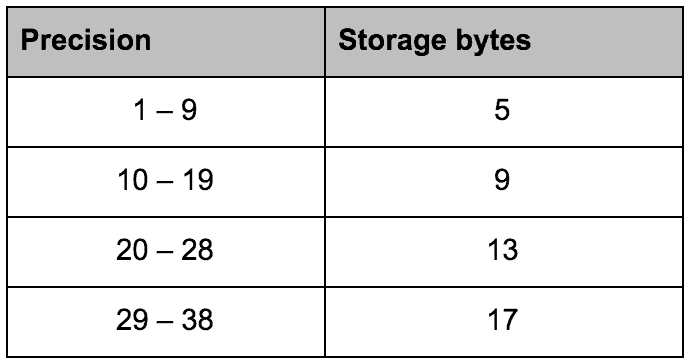Arithmetic overflow error; converting it to data type numeric
Understanding Decimal and Numeric arguments

## The problem:

Entering a number in a field that is data type of decimal (5, 3) works for values like [85.200] but not others like .

## The Cause:

The arguments for decimal and numeric data types are (p, s) where ‘p’ stands for precision and the ‘s’ stands for scale. Combined, these criteria enforce the range of values that may be entered. According to the Microsoft documentation, the precision criteria prescribes how many digits the value can have in total. This total is regardless of whether the digits sit to the left or the right of the decimal point. The maximum value for precision is 38.

The scale prescribes two additional aspects of the field’s value. First, it sets how many of the total digits are reserved for the right side of the decimal point. Second, as a byproduct of the first, it limits the maximum number of digits that can be stored to the left of the decimal point. The default arguments for decimal and numeric data types are (18, 0).

## The Solution:

Applying this to the problem described we can see that the reason why the value  creates an error is that the numeric data type has only been assigned 5 total digits and 3 of them are reserved for the right side of the decimal leaving only 2 available for the left side. This limitation is strictly for the left side of the decimal so other values such as [5.1234] will not cause an error because we are still within the datatype precision of 5 digits. Any value larger than [99.999] will not be a valid input for this field under these conditions. To correct this problem the arguments would need to be changed accordingly:

• From decimal (5, 3) – To decimal (6, 3)

Performing this correction will allow values ranging from [0.0001] to [999.999] to be entered in the field. Of course, doing so will have a storage impact, which is based on the precision. The chart below shows the storage sizes for the various precision argument ranges.